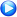# Compare Origin and OriginPro

## Introduction

OriginPro includes all of the features available in Origin. In addition OriginPro provides advanced analysis tools and Apps. The following tables summarize the key benefits of OriginPro by feature areas.

For a broad overview of the features in both products, please visit our Origin product page.

This information is also available as a PDF file.

## Comparison of Origin and OriginPro

Not all features are shown in the following tables. If you don't see a feature listed here, you can assume that it exists in both regular Origin and OriginPro.

### Curve Fitting

Curve Fitting Origin OriginPro
Linear and Polynomial Fitting
Linear Regression
Linear Fit with X Error
Confidence Ellipse for Linear Fit
Polynomial Regression
Multiple Linear Regression
Partial Leverage Plots in Multiple Regression
Residual Analysis
Nonlinear Fitting
Fitting Multiple Datasets
Built-in Fitting Function and User-Defined Fitting Function
Parameter Initialization and Derived Parameter Definition
Bounds and Constraints
Weighted Fitting
Fitting with Y Error
Fitting with X and Y Errors (Orthogonal Regression)
Global Fit with Parameter Sharing
Fitting Replica Data
Residual Analysis
Fitting with Implicit Functions (Orthogonal Distance Regression)
Fitting Comparison
Fit and Rank Multiple Models
Surface Fitting

### Peak Analysis

Peak Analysis Origin OriginPro
Peak Analysis
Baseline Detection
Baseline Subtraction
Peak Finding
Peak Integration
Peak Fitting
Fit Baseline with Peaks
Fit Individual Peaks with Different Fitting Functions
Batch Peak Analysis

### Statistics

Statistics Origin OriginPro
Descriptive Statistics
Basic Descriptive Statistics
1D and 2D Frequency Counts
Correlation Coefficient
Partial Correlation Coefficient
Cross Tabulation
Discrete Frequency
Distribution Fit
Normality Test (Shaprio-Wilk, Lilliefors, Kolmogorov-Smirnov, Anderson-Darling, D'Agostino-K Squared, Chen-Shapiro)
Statistics Charts: Histogram, Box Chart, Scatter Matrix, QC Chart, Probability Plot, Q-Q Plot, and Pareto Chart
Grubbs Test and Q-test to Detect Outliers
Hypothesis Testing
One Sample and Two-Sample t-Test, Pair-Sample t-Test
Two Sample and Paired-Sample T-Test on Rows
One Sample and Two Sample Hypothesis Tests for Variance
One and Two-Proportion Test
Analysis of Variance
One Way ANOVA, Two Way ANOVA
Three Way ANOVA
ANOVA: Mean Comparison (Tukey, Bonferroni, Scheffe, Dunn-Sidak, Fisher LSD, Holm-Bonferroni, Holm-Sidak)
One Way and Two Way Repeated Measure ANOVA
Nonparametric Tests
Sign Test
Wilcoxon Test for One Sample and Paired Sample
Two Sample Kolmogorov-Smirnov Test
Mann-Whitney Test
Kruskal-Wallis ANOVA
Mood's Median Test
Friedman ANOVA
Multivariate Analysis
Principal Component Analysis
Cluster Analysis
Discrimininant Analysis
Canonical Discriminant Analysis
Partial Least Squares
Survival Analysis
Kaplan-Meier Estimator
Test Equality of Survival Functions (Log-Rank, Breslow and Tarone-Ware )
Cox Proportional Hazard Model
Weibull Fit
Power and Sample Size
One, Two and Paired-Sample t-Test,One Way ANOVA, One and Two-Proportion Test, One and Two -Variance Test
ROC Curve
ROC Curve

### Signal Analysis

Signal Analysis Origin OriginPro
Smoothing and Filtering
Smoothing using Savitzky-Golay Filter, Adjacent Averaging, FFT Filter, Percentile Filter
FFT Filters: Low Pass, Low Pass Parabolic, High Pass, Band Pass, Band Block, and Threshold
IIR Filter Design
Fast Fourier Transform (FFT)
FFT with Basic Options
2D FFT and 2D IFFT Basic Filtering
Short-Time Fourier Transform (STFT)
Wavelet Analysis
Discrete Wavelet Transform (DWT) and Inverse Discrete Wavelet Transform (IDWT)
Wavelet Smoothing
Wavelet DeNoising
Continuous Wavelet Transform (CWT)
Evaluation of Continuous Wavelet Function
Others
Convolution and Deconvolution
Coherence
1D Correlation
2D Correlation
Hilbert Transform
Signal Envelope
Signal Decimation
Rise and Fall Time Analysis

### Mathematics

Mathematics Origin OriginPro
Simple Mathematics Operations
Simple Mathematics Operations on or Between Datasets
Set Cell, Column or Matrix Values by Using Mathematics Operations
Normalization
Interpolation and Extrapolation
1D Interpolation and Extrapolation
Interpolation and Extrapolation of Y From X
Trace Interpolation on XY Data
Trace Interpolation on XYZ Data
2D Interpolation and Extrapolation
3D Interpolation
Differentiation and Integration
Numerical Differentiation
1D Numerical Integration
2D Volume Integration
Area Calculation
Polygon Area
XYZ Surface Area
Matrix Surface Area
Others
Average Multiple Curves
Inverse of a Matrix

### Gadget

Gadget Origin OriginPro
Gadget
Surface Integration Gadget
Global Vertical Cursor Gadget Across Graphs
Intersect Gadget
Quick Sigmoidal Fit Gadget
Cluster Gadget
Quick Peaks Gadget
Differentiate and Interpolate Gadget
Quick Fit Gadget
Rise Time Gadget
Integrate, FFT and Statistics Gadget

### Apps

Apps Origin OriginPro
Curve Fitting
Quantile Regression
Fit ODE
Speedy Fit
Global Fit with Multiple Functions
3D SmootherFit Convolution
Rank Models
Constrained Multiple RegressionPolynomial Surface FitSequential FitPiecewise FitEnzyme Kinetics
General Linear Regression
Composite Spectrum RegressionCyclic Voltammetry
Find a Fitting Function
2D SmootherSimple FitCompare Datasets
Compare Linear Fit Parameters and Datasets
Peak Analysis
Gel Molecular Weight Analyzer
Global Peak Fit
Peak Deconvolution
Align Peaks
Mathematics
Equation Solver
Concave Hull
Curvature Radius
Tangent
Tafel Extrapolation
Distance Between Two Points
Level CrossingSignal Processing
Independent Component Analysis
Dynamic Time Warping
Simple pCLAMP Analyzer
Time-Frequency Analysis
Fourier Self-Deconvolution
FFT Examiner
White Noise
Statistics
2D Correlation Spectroscopy Analysis
Change Point Analysis
Design of Experiments
Gaussian Mixture Models
Factor Analysis
Simple Time Series Analysis
Logistic Regression
Principal Component Analysis for Spectroscopy
Advanced Principal Component Analysis
Hotelling's T-squared Test
Post-hoc Analysis for Nonparametric Tests
Hurst Exponent
Statistics Advisor
Graph Customization
Color EditorLaTeXGoogle Map ImportMaps OnlineImport ShapefileColormap for Map Data
Layer Stack Manager
Graphing
Means Plot
Manhattan Plot for GWAS (Genome-Wide Association Studies)
Image Stack Profile
Heatmap with Dendrogram
3D Wind Rose
Isosurface
Graph Maker
Batch Plotting
Voronoi-Diagram
Chromaticity Diagram
Paired Comparison PlotKernel Density for Polar and Ternary3D Wall Profile
Plot Sub MatrixLog Histogram
Zoomed Inset
Treemap Plot
Data Processing
Distributed Batch ProcessingHysteresis
OPJ Packer
Toolbar MakerData SlicerOPJ SearcherImport and Export
Import Chem Data
Import SAS XPT Files
Graph Publisher
Import LSM
Movie Creator
HDF5 Browser
Import Tektronix WFM Files
Agilent MS ReaderSend Graphs to PowerpointSend Graphs to WordNote: New Apps are released frequently. Please visit originlab.com/Apps for the most up-to-date list. OriginPro-only Apps will be marked with the PRO symbol.

Note: If you don't see features listed here, it means they exist in both regular and Pro

 © OriginLab Corporation. All rights reserved.